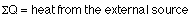## Heat

12-3-99

Sections 14.1 - 14.6

### Temperature, internal energy, and heat

The temperature of an object is a measure of the energy per molecule of an object. To raise the temperature, energy must be added; to lower the temperature, energy has to be removed. This thermal energy is internal, in the sense that it is associated with the motion of the atoms and molecules making up the object.

When objects of different temperatures are brought together, the temperatures will tend to equalize. Energy is transferred from hotter objects to cooler objects; this transferred energy is known as heat.

### Specific heat capacity

When objects of different temperature are brought together, and heat is transferred from the higher-temperature objects to the lower-temperature objects, the total internal energy is conserved. Applying conservation of energy means that the total heat transferred from the hotter objects must equal the total heat transferred to the cooler objects. If the temperature of an object changes, the heat (Q) added or removed can be found using the equation: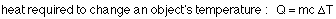where m is the mass, and c is the specific heat capacity, a measure of the heat required to change the temperature of a particular mass by a particular temperature. The SI unit for specific heat is J / (kg °C).

This applies to liquids and solids. Generally, the specific heat capacities for solids are a few hundred J / (kg °C), and for liquids they're a few thousand J / (kg °C). For gases, the same equation applies, but there are two different specific heat values. The specific heat capacity of a gas depends on whether the pressure or the volume of the gas is kept constant; there is a specific heat capacity for constant pressure, and a specific heat capacity for constant volume.

### Example

0.300 kg of coffee, at a temperature of 95 °C, is poured into a room-temperature steel mug, of mass 0.125 kg. Assuming no energy is lost to the surroundings, what does the temperature of the mug filled with coffee come to?

Applying conservation of energy, the total change in energy of the system must be zero. So, we can just add up the individual energy changes (the Q's) and set the sum equal to zero. The subscript c refers to the coffee, and m to the mug.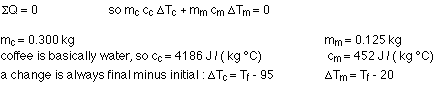Note that room temperature in Celsius is about 20°. Re-arranging the equation to solve for the final temperature gives: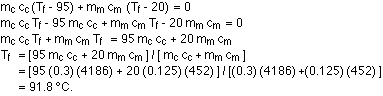The temperature of the coffee doesn't drop by much because the specific heat of water (or coffee) is so much larger than that of steel. This is too hot to drink, but if you leave it heat will be transferred to the surroundings and the coffee will cool.

### Changing phase; latent heat

Funny things happen when a substance changes phase. Heat can be transferred in or out without any change in temperature, because of the energy required to change phase. What is happening is that the internal energy of the substance is changing, because the relationship between neighboring atoms and molecules changes. Going from solid to liquid, for example, the solid phase of the material might have a particular crystal structure, and the internal energy depends on the structure. In the liquid phase, there is no crystal structure, so the internal energy is quite different (higher, generally) from what it is in the solid phase.

The change in internal energy associated with a change in phase is known as the latent heat. For a liquid-solid phase change, it's called the latent heat of fusion. For the gas-liquid phase change, it's the latent heat of vaporization, which is generally larger than the latent heat of fusion. Latent heats are relatively large compared to the heat required to change the temperature of a substance by 1° C.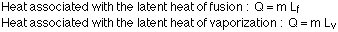If you use the sum-of-all-the-Q's equals zero equation, you have to be careful with the heat associated with something changing phase because you need to put it in with the appropriate sign. If heat is going into a substance changing phase, such as when it's melting or boiling, the Q is positive; if heat is being removed, such as when it's freezing or condensing, the Q is negative. We don't have to worry about the signs for the heat required to change temperature, because the sign is already built in to the change in temperature.

The textbook use an alternate approach (see example 14-5 on page 422). In that method, all the heat losses are set equal to all the heat gains, with everything (even the changes in temperature) going in with positive signs.

Note that a change in phase takes place only under the right conditions. Water, for example, doesn't freeze at 10 °C, at least not at atmospheric pressure. If you had water at that temperature, you would first need to cool it to the melting point, 0 °C, before it would start to freeze.

If you're putting in heat from an outside source, the sum-of-all-the-Q's equation becomes: